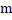# An artificial satellite is moving in a circular orbit around the earth with a speed equal to the escape velocity from the earth of radius R. Then what is the height of the satellite above the surface of the earth? (a) R/2 (b) R (c) 3 R (d) None of these

## Question ID - 152052 :- An artificial satellite is moving in a circular orbit around the earth with a speed equal to the escape velocity from the earth of radius R. Then what is the height of the satellite above the surface of the earth? (a) R/2 (b) R (c) 3 R (d) None of these

3537

None of these

Next Question :
 The mass of earth is 80 times that of moon and its diameter is double that of moon. If the value of acceleration due to gravity on earth is 9.8 m s-2 , then the value of acceleration due to gravity on moon will be (a)s-2 (b)s-2 (c) 9.8s-2 (d) 4.9s-2